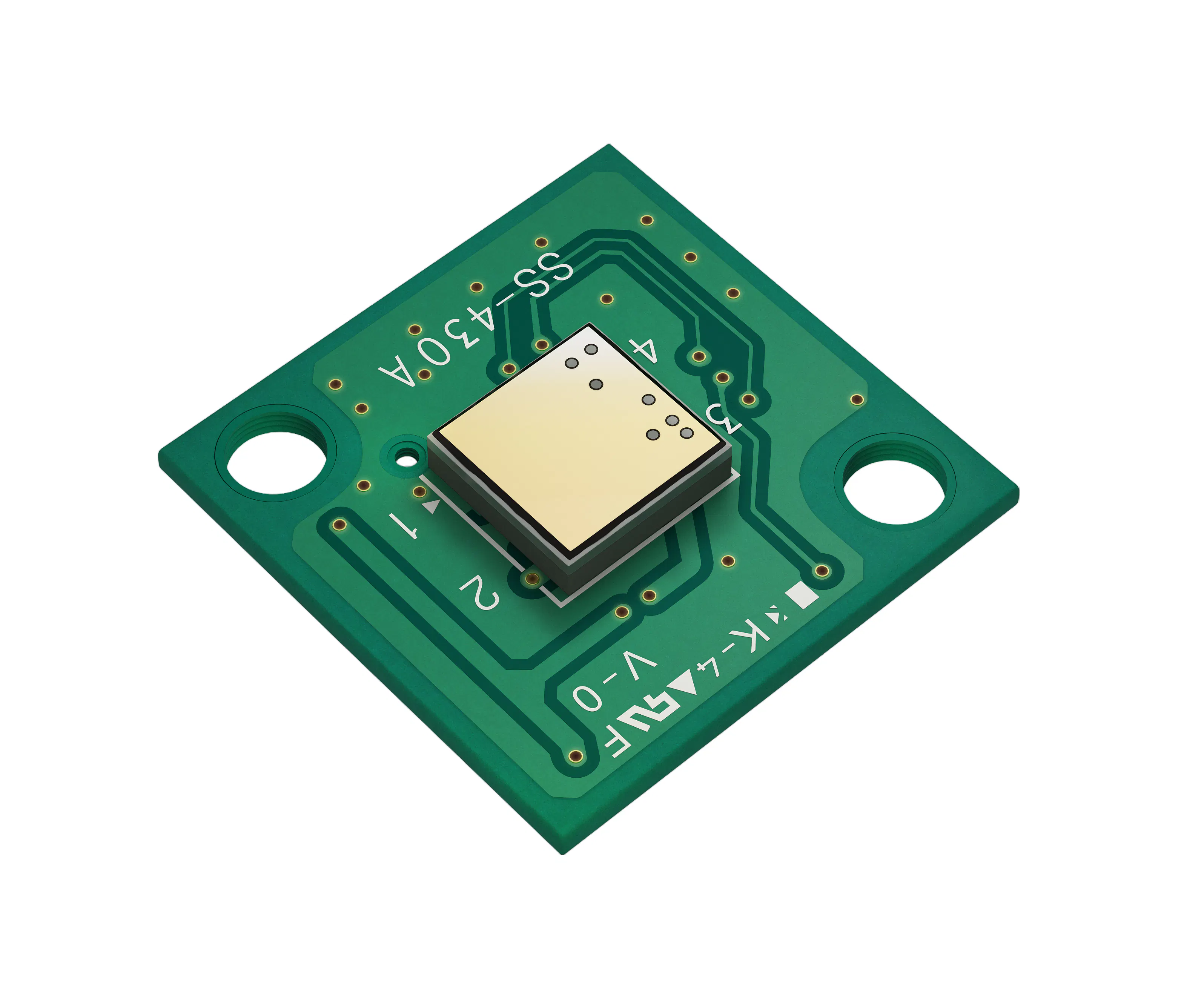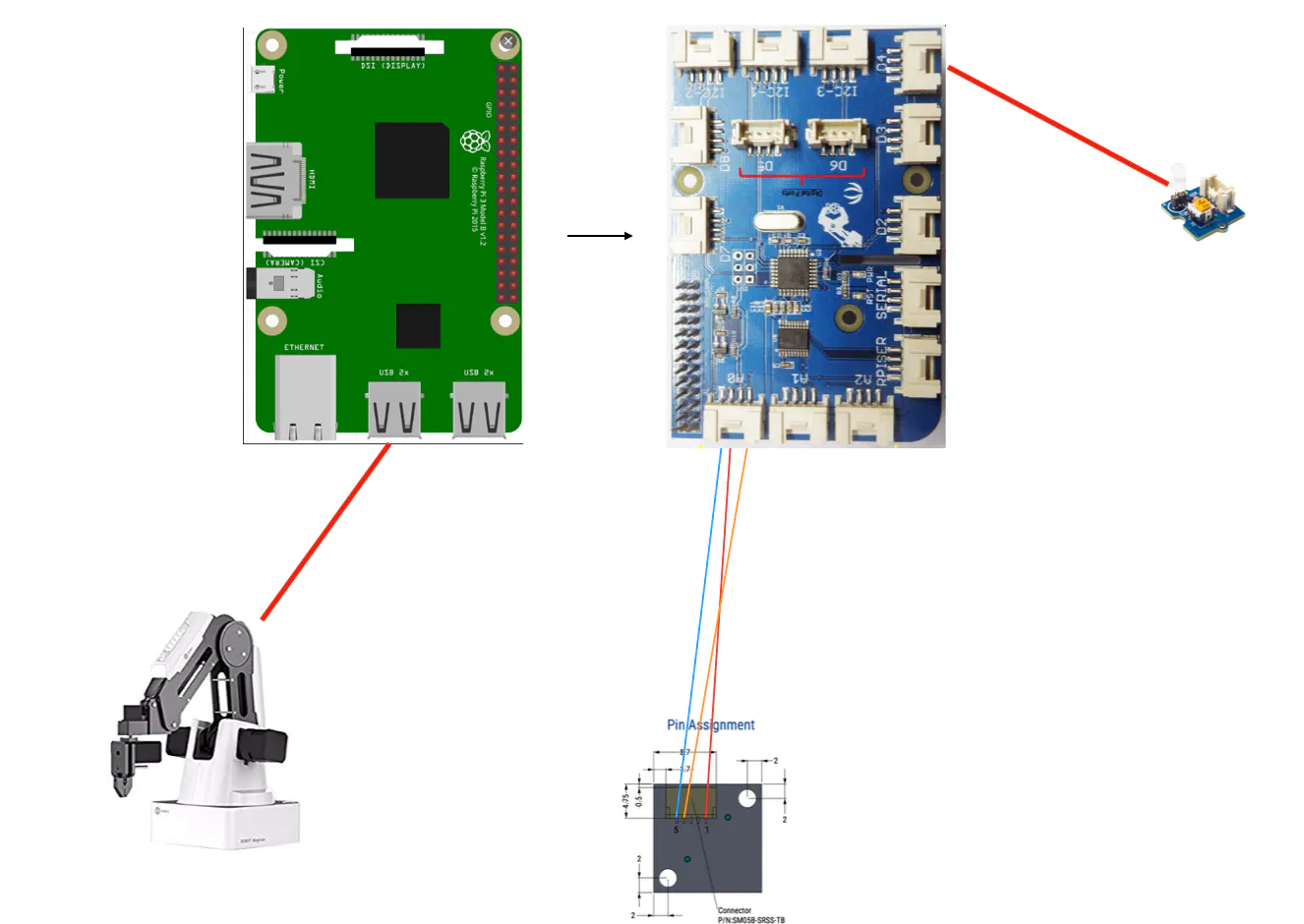# Proximity Candy Bot

Our robotic arm will automatically welcome our guests with candies

IntermediateFull instructions provided16 hours748

## Things used in this project

### Hardware componentsKEMET Electronics Corporation Proximity Sensor- Pyroelectric Infrared Sensor Module
×1Raspberry Pi 3 Model B
×1
 Seeed Grove Shield for Pi
×1
 Dobot Magician
×1

### Hand tools and fabrication machines3D Printer (generic)

## Schematics

### Candy bot robotic arm schema

Candy bot robotic arm schema## Code

### candybot.py

Python
candybot file
```import time
import os
import grovepi
from grovepi import *
from datetime import datetime

cred = credentials.Certificate("/path/to/your_account-service_key")

# Initialize the app with a service account, granting admin privileges
'databaseURL': 'https://your_candy_bot.firebaseio.com/'
})

ref = db.reference('/candy')

#Sensor connected to A0 Port
sensor = 14		# Pin 14 is A0 Port.
led = 4

grovepi.pinMode(sensor,"INPUT")
pinMode(led,"OUTPUT")

count = 0
last_value = 0

while True:
try:
print ("sensor_value = %d" %sensor_value)

if sensor_value > 200:
count += 1

if sensor_value <= 200 and last_value > 200:
count = 0 #reset the count

if count > 2:
count = 0
digitalWrite(led,1)		# Send HIGH to switch on LED
print('called robotic arm')
os.system('python3 Script.py --Json candybot.json')
digitalWrite(led,0)		# Send LOW to switch off LED
ref.set({'count': 1, 'time': datetime.now().strftime("%d-%b-%Y (%H:%M:%S.%f)")})
last_value = sensor_value

time.sleep(.5)

except IOError:
print ("Error")
```

### Dobot Magician Scirpt

Python
script to control the DobotMagician
```import threading
import DobotDllType as dType
import time
import argparse
import json
from pprint import pprint
from collections import OrderedDict

class ScriptRobot():

global CON_STR
CON_STR = {
dType.DobotConnect.DobotConnect_NoError:  "DobotConnect_NoError",
dType.DobotConnect.DobotConnect_NotFound: "DobotConnect_NotFound",
dType.DobotConnect.DobotConnect_Occupied: "DobotConnect_Occupied"}

def __init__(self,Json):
self.Json=Json
self.state=""

def Connect(self):
#Connect Dobot
self.state = dType.ConnectDobot(self.api, "", 115200)
dType.GetDeviceSN(self.api)
dType.GetDeviceName(self.api)
dType.GetDeviceVersion(self.api)
dType.GetDeviceWithL(self.api)
dType.GetPoseL(self.api)
dType.GetKinematics(self.api)
#dType.GetHOMEParams(self.api)
print("Connect status:",CON_STR[self.state])
if (self.state == dType.DobotConnect.DobotConnect_NoError):
dType.SetQueuedCmdClear(self.api)
return True
else :
dType.DisconnectDobot(self.api)
return False
"""
def _MOVJ(self,data):
dType.SetPTPCmd(self.api,dType.PTPMode.PTPMOVJXYZMode,float(data['X']),float(data['Y']),float(data['Z']),float(data['R']), isQueued = 1)

def _MOVL(self,data):
dType.SetPTPCmd(self.api,dType.PTPMode.PTPMOVLXYZMode,float(data['X']),float(data['Y']),float(data['Z']),float(data['R']), isQueued = 1)
def _ARC(self,value,data):
dType.SetARCCmd(self.api,[float(data['X']),float(data['Y']),float(data['Z']),0],[float(data['_X']),float(data['_Y']),float(data['_Z']),0], isQueued = 1)
def moveTypes(self,value,data):
if value=="MOVJ" :
return self._MOVJ(data)
elif value=="MOVL" :
return self._MOVL(data)
elif value=="ARC" :
return self._ARC(value,data)
"""
def ParserMove(self):
dType.SetQueuedCmdClear(self.api)
json_data = open(self.Json)
#def SetPTPCoordinateParams(api, xyzVelocity, xyzAcceleration, rVelocity,  rAcceleration,  isQueued=0):

for move in data:
#print "TEST_:"+data[move]['MotionStyle'],data[move]
if data[move]['MotionStyle']=="MOVJ" :
lastIndex=dType.SetPTPCmd(self.api,dType.PTPMode.PTPMOVJXYZMode,float(data[move]['X']),float(data[move]['Y']),float(data[move]['Z']),float(data[move]['R']), isQueued = 1)
dType.SetEndEffectorGripper(self.api, data[move]["Enabled"], data[move]['Gripper'], isQueued= 1)
print(data[move]["Gripper"])
if data[move]['PauseTime']!=0:
lastIndex=dType.SetWAITCmd(self.api,float(data[move]['PauseTime']), isQueued = 1)
if data[move]['MotionStyle']=="MOVL" :
lastIndex=dType.SetPTPCmd(self.api,dType.PTPMode.PTPMOVLXYZMode,float(data[move]['X']),float(data[move]['Y']),float(data[move]['Z']),float(data[move]['R']), isQueued = 1)
if data[move]['MotionStyle']=="MOVJANGLE" :
lastIndex=dType.SetARCCmd(self.api,[float(data[move]['X']),float(data[move]['Y']),float(data[move]['Z']),0],[float(data[move]['_X']),float(data[move]['_Y']),float(data[move]['_Z']),0], isQueued = 1)

dType.SetQueuedCmdStartExec(self.api)

while lastIndex > dType.GetQueuedCmdCurrentIndex(self.api):
#           dType.GetPose(self.api) Obtener la Posicion actual del robot
dType.dSleep(100)

dType.SetQueuedCmdStopExec(self.api)
dType.GetKinematics(self.api)

dType.DisconnectDobot(self.api)

if __name__ == "__main__":
parser = argparse.ArgumentParser(description='Script Robot')
parser.add_argument('--Json', required=True, help='File name export json')
args = parser.parse_args()
R = ScriptRobot(args.Json)
R.Connect()
R.ParserMove()
```

### Firebase test code

Python
Fire base test code
```from datetime import datetime

cred = credentials.Certificate("/path/to/your_account-service_key")

# Initialize the app with a service account, granting admin privileges
'databaseURL': 'https://your_candy_bot.firebaseio.com/'
})

ref = db.reference('/candy')
ref.set({'count': 1, 'time': datetime.now().strftime("%d-%b-%Y (%H:%M:%S.%f)")})
```

### Kemet Candy Bot repo

Kemet Candy Bot repo

## Credits

### Peter Ma

16 projects • 293 followers
Prototype Hacker, Hackathon Goer, World Traveler, Ecological balancer, integrationist, technologist, futurist.